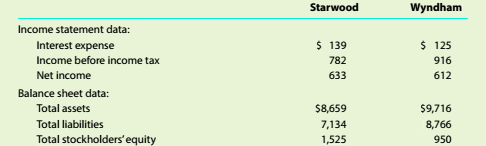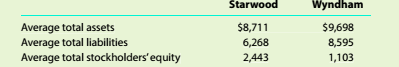Chapter 9, Problem 9.5.2C

Chapter
Section
Textbook Problem

Comprehensive profitability and solvency analysis Starwood Hotels & Resorts Worldwide Inc. (HOT) and Wyndham Worldwide Corporation (WYN) are two major owners and managers of lodging and resort properties in the United States. Financial data (in millions) for a recent year for the two companies are as follows:The average liabilities, stockholders' equity, and total assets were as follows:Analyze and compare the two companies, using the information in (1).

To determine

Introduction:

Accounting ratios are used to evaluate the financial performance of the business organisation

Profitability analysis:

Itis used to evaluate the company's ability to generate income relative to revenue, assets, operating cost and shareholders' equity during a specific period. It measures how efficiently the resources have been utilised to generate profit.

Solvency analysis:

It is used to evaluate companies' ability to pay its long-term debt, it also helps owner to determine the chances of firm's long-term survival.

To analyze:

The derived ratios.

Explanation
 Ratios S Co. W Co. Return on total assets 633+139 8,711=0.08 612+125 9,698=0.08 Return on shareholder's equity 633 1525=0.42 612 950=0.64 Times interest earned 782+139 139=6.63 916+125 125=8.33 Debt ratio 7,1348,659=0.82 8,7669,716=0.90 Debt to equity ratio 7,1341,525=4

Still sussing out bartleby?

Check out a sample textbook solution.

See a sample solution

The Solution to Your Study Problems

Bartleby provides explanations to thousands of textbook problems written by our experts, many with advanced degrees!

Get Started

Find more solutions based on key concepts